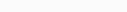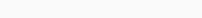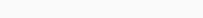# Reasoning Hard Questions Test

Reasoning Hard Questions Test : Number set analogy Questions from SSC CHSL, CGL Exam Paper. Questions with Answers and solution is very useful for upcoming competitive examinations.

## Numbers Set Analogy Test:1

Number of Questions : 15
Time : 15 Minutes

Select the option in which the numbers are related in the same way as are the numbers of the following set.
उस विकल्प का चयन करें, जिसमें संख्याएँ उसी प्रकार संबंधित हैं, जिस प्रकार निम्न समुच्चय (सेट) की संख्याएँ संबंधित हैं।

Q. 1: {9, 12, 18}
a) {5, 12, 24}
b) {13, 19, 31}
c) {21, 29, 44}
d) {7, 13, 27}

Ans: b) {13, 19, 31}
Solution: 12 – 9 =3 and 18-12 =6, Difference is double
Same manner in option (b)
19-13 =6 and 31-19 =12
In other option this rule is not applicable

Q.2: Select the option in which the numbers are related in the same way as are the numbers of the following set.
(42, 14, 294)
a) (30, 16, 242)
b) (34, 18, 306)
c) (21, 15, 205)
d) (36, 21, 360)

Ans : b) (34, 18, 306)
Solution:$42\times14 \div 2 = 294$
and$34 \times 18 \div 2 =306$
Others options are not following this rule.

Q.3: Select the option in which the numbers are related in the same way as are the numbers of the following set.
(4, 3, 73)
a) (7, 5, 345)
b) (5, 4, 141)
c) (6, 2, 230)
d) (5, 7, 302)

Ans : b) (5, 4, 141)
Solution: 43 + 32 =64+9 =73
53+42= 125+16 = 141

Q.4: Select the option in which the numbers share the same relationship as that shared by the given pair of numbers.
185 : 199

a) 139 : 152
b) 174 : 187
c) 126 : 134
d) 89 : 106

Ans : d) 89 : 106
Solution: 1+8+5 = 14, 185 +14 = 199
Option (d) 8+9 = 17, 89+17 = 106

Q.5: Select the option in which the numbers are related in the same way as are the numbers of the following set.
(2194, 1328, 726)

a) (1170, 570, 228)
b) (1560, 1131, 551)
c) (1406, 962, 390)
d) (1725, 997, 509)

Ans : d) (1725, 997, 509)
Solution:
133 = 2197, 2197-3 =2194,
113 = 1331, 1331-3 =1328,
93 = 729, 729-3 =726,
Same way
123=1728 , 1728-3=1725
103=1000, 1000-3 =997
83= 512, 512-3=509

Q.6: Select the option in which the numbers are related in the same way as are the numbers of the following set.
47 : 58 : 71

a) 72 : 81 : 90
b) 89 : 98 : 106
c) 76 : 89 : 105
d) 68 : 80 : 88

Ans : a) 72 : 81 : 90
Solution:
47 + (4+7) =58
58 + (5+8) = 71
Same way
72 +(7+2) = 81
81+(8+1)=90

Q.7: Select the option that is related to the third number in the same way as the second number is related to the first number.
18 : 163 : : 24 : ?

a) 216
b) 289
c) 298
d) 222

Ans : b) 289
Solution:$18^2=324, 324 \div2+1 =163$
Same way$24^2=576, 576 \div2+1 =289$

Q.8: Select the option in which the numbers share the same relationship as that shared by the given pair of numbers.
12 : 20

a) 30 : 42
b) 24 : 36
c) 42 : 57
d) 18 : 28

Ans : a) 30 : 42
Solution:
3×4 =12 : 4×5=20
Same way
5×6 =30 : 6×7=42

Q.9: Select the option in which the numbers are related in the same way as are the numbers of the following set.
(72, 56, 42)

a) (80, 48, 24)
b) (132, 110, 90)
c) (80, 68, 58)
d) (126, 102, 80)

Ans : b) (132, 110, 90)
Solution:
9×8=72, 8×7=56, 7×6=42
Same way
12×11=132, 11×10=110, 10×9=90

Q.10: Select the option in which the numbers are related in the same way as are the numbers of the following set.
(21, 13, 17)

a) (11, 21, 140)
b) (25, 35, 38)
c) (22, 57, 81)
d) (32, 46, 39)

Ans : d) (32, 46, 39)
Solution:
21+13=34, 34/2 =17
Same way
32+46 = 78, 78/2 =39

Q.11: Select the option in which the numbers in the set share the same relationship as the numbers in the given set.
(25, 19, 36)

a) (27, 11, 38)
b) (20, 14, 66)
c) (24, 22, 49)
d) (22, 17, 25)

Ans : d) (22, 17, 25)
Solution:
25-16=6, 62=36
Same way
22-17=5, 52=25

Q.12: Select the option in which the numbers in the set share the same relationship as the numbers in the given set.
(14, 22, 77)

a) (20, 16, 105)
b) (17, 15, 76)
c) (18, 32, 144)
d) (22, 11, 86)

Ans : c) (18, 32, 144)
Solution:
14×22=308, 308/4 =77
Same way
18×32=576, 576/4=144

Q.13: Select the option in which the numbers share the same relationship as that shared by the given pair of numbers.
28 : 70

a) 18 : 45
b) 12 : 32
c) 30 : 84
d) 34 : 86

Ans : a) 18 : 45
Solution:
28X2.5=70
Same way
18×2.5=45

Q.14: Select the option in which the numbers are related in the same way as are the numbers of the following set.
{2, 2, 9}

a) {8, 36, 81}
b) {6, 18, 46}
c) {4, 8, 25}
d) {12, 60, 98}

Ans : c) {4, 8, 25}
Solution:
2+2 = 4, 4×2+1=9
Same way
4+8=12, 12×2+1=25

Q.15: Select the set in which the numbers are related in the same way as are the numbers of the following set.
(2, 6, 9)

a) (4, 12, 18)
b) (8, 12, 17)
c) (5, 15, 30)
d) (7, 22, 17)

Ans : a) (4, 12, 18)
Solution:
2×3=6, 6×1.5=9
Same way
4×3=12. 12×1.5=18

Thanks for attempt this Reasoning Hard Questions Test on Number sets analogy.

For More Reasoning Hard Questions Test – Numbers Set Analogy Test 2

### Reasoning Questions Practice Set

Reasoning Questions Mock Test
Questions : 25

## Reasoning Topic wise Question – Mock Test

### 1 thought on “Reasoning Hard Questions Test”

1.Rohit Prajapati

Q4 option 1 also can be correct 👍 1+3+9=13, 139+13=152

Scroll to Top